# Tablecloths Size Guide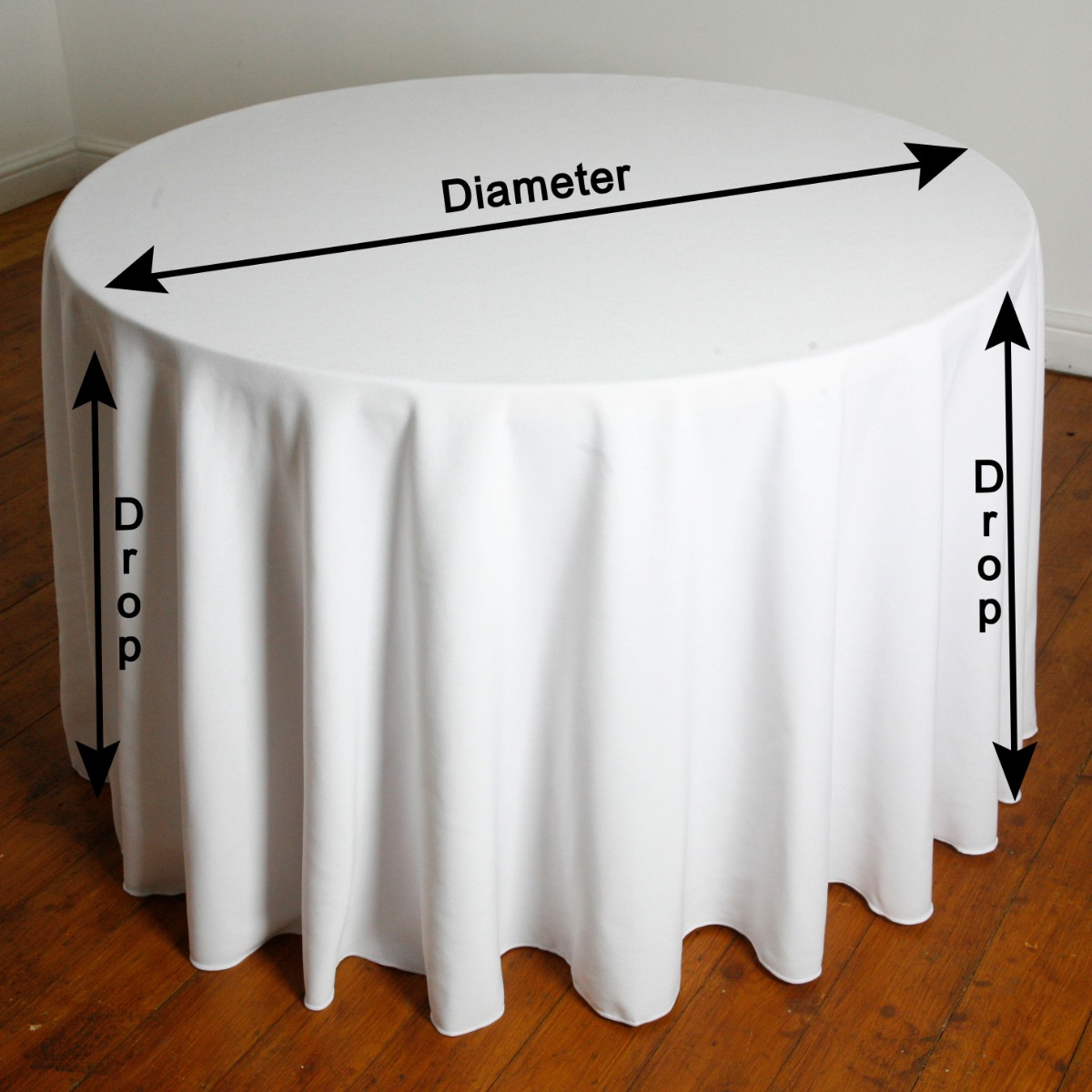# What Size Tablecloth Do I Need?

Step 1

Find a pen, pad & measuring tape

Measure your table top surface size, width & length for square & rectangular tables

Measure your table top surface diameter for round tables

Draw a sketch of your table with its dimensions as a visual aid, to help work out the correct size tablecloth required

The use of a calculator may also be helpful

Step 2

*All items on the website are the actual tablecloth sizes

Don't forget, to calculate the correct size tablecloth for your table, allow for the tablecloth over hang, to drop down from the top of the table

For square & rectangular tables, add extra width & length for each of the 2 sides & 2 ends of the table measurement, for the cloth drop required down the table

For round tables, add the extra length required twice to the table diameter measurement, for the cloth to drop evenly all around the table

A standard height of a table is 29" - 30"

To convert inches to cm multiply by 2.54  e.g. 1" x 2.54 = 2.54cm

To covert feet to inches muiltiply by 12   e.g 1 foot x 12 = 12 inches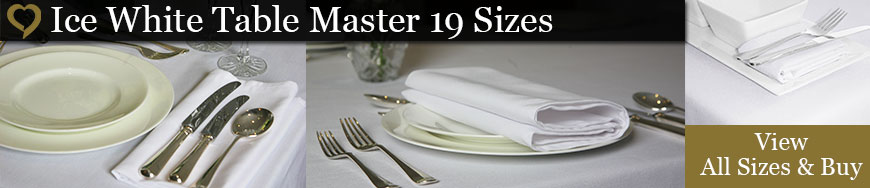Step 3

Size guide charts - square. rectangular & circular tablecloths

A) Find your table size in the left-hand side column of the chart - Table Size

B) View the various options from left to right, which shows how much the tablecloth will drop down the table

C) Finally, look at the top bar of the chart which shows the tablecloth size required - Tablecloth Sizes

The Drop is the how far the tablecloth will drop down from the top of the table

The Clearance is the distance between the end of the tablecloth drop down the table, to the floor ( The tablecloth does not cover the table in the clearance )

* Guides below are based on UK standard size tables, standard height of a table is 29" - 30" ( 74cm -76cm )Example - Square Table - Width & Length Of The Table Top

Square table 3ft wide x 3ft length = 36" x 36" or (91cm x 91cm)

This is a standard restaurant square table size

A tablecloth size of 52" x 52" will give a drop of 8" down each side.

* A standard height of a table is 29" - 30"

Calculation for 36" x 36" square table

(Tablecloth size minus table size) then divide by 2

E.g. (52” minus 36”) = 16 then divide by 2 = 8” drop on all sides of the table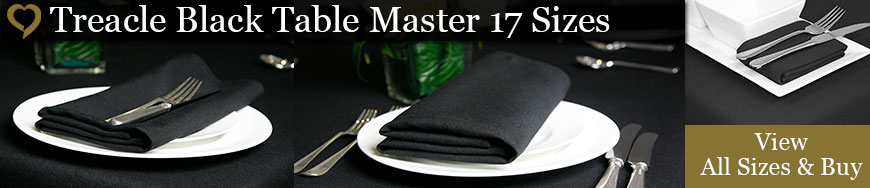Example - Rectangular Table -  Width & Length Of The Table Top

Rectangular table 2ft 6"wide x 6ft length  =  30" x 72" This is a standard trestle table size

A tablecloth size of 70" x 108" will give a drop of 20" down each side and 18" down each end

* A standard height of a table is 29" - 30"

Calculation for  30" x 72" rectangle table

W (Tablecloth width size minus table width size) then divide by 2

L (Tablecloth length size minus table length size) then divide by 2

E.g.

W (70” minus 30”) = 40 then divide by 2 = 20” drop on each side of the table

L (108” minus 72”) = 36 then divide by 2 = 18” drop at each end of the tableExample - Circular Round Table 6ft / 72" Diameter Of The Table Top Surface

A tablecloth size of 88" will give a drop around the table of 8" around the table

A tablecloth size of 108" will give a drop around the table of 18" around the table

A tablecloth size of 118" will give a drop around the table of 23" around the table

A tablecloth size of 130" will give a drop around the table of 29" around the table [Full coverage]

* A standard height of a table is 29"- 30"Calculation Methods For Circular Round Tablecloth Size Required

Measure the table top surface diameter of your table.

Then Add Twice the required tablecloth drop down the table.

For example a 72 inch round table top surface diameter, with a 29 inch tablecloth drop down the table required, would need a 130 inch round tablecloth

Calculated as 72 + 29 + 29 = 130

* A standard height of a table is 29"- 30"

Or use the method below, which is a bit more complex.

(Tablecloth Size Diameter) Minus (Diameter Of The Table Top Surface) Then Divide By 2 = The Drop Around The Table

E.g. Tablecloth size diameter 130" (Minus) diameter of the table top surface 72" = 58"

Then 58" divide by 2 = 29" tablecloth drop around the table

Have we confused you with the tablecloth calculations & examples?

We are here to help on what is the correct size tablecloth you require?

Double check to avoid disappointment when your tablecloth order arrives

Find a pen, pad & measuring tape

Send your measurements & request via the message box and we will check or suggest the correct size for you.

Or

When you have your table size measurements details, please call us on Free Phone 0800 612 1379

If you have access to the website, we will also guide you to the appropriate information & product pages

#### (Sales Lines Open Monday-Friday 9:00am-5:00pm)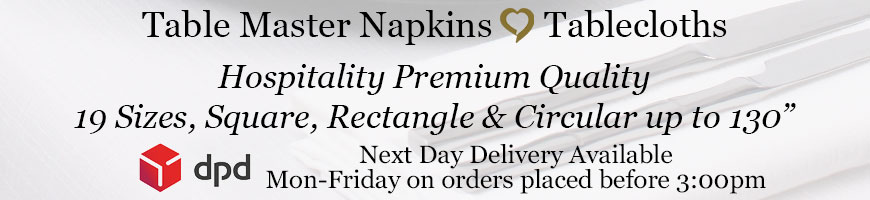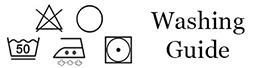## Ideal for Hospitality, Hotels, Restaurants, Healthcare and the Home.

The tablecloths are absorbent, soft and durable with a texture ‘Cotton Feel’ touch.

"Love Tablecloths" polyester tablecloths are strong, durable with low colour loss and minimal shrinkage. Made with 100% polyester vortex air spun textured, blended and twisted yarns. The tablecloths are woven, dyed and finished on state of the art machinery, given reinforced hems and high quality stitching to protect the longevity of the tablecloths.

Quick Overview

Wash at 50°C with a biological detergent - Do not overload the washing machine - Short spin cycle - Hang dry or low temp tumble dry - Medium temperature iron setting with steam.